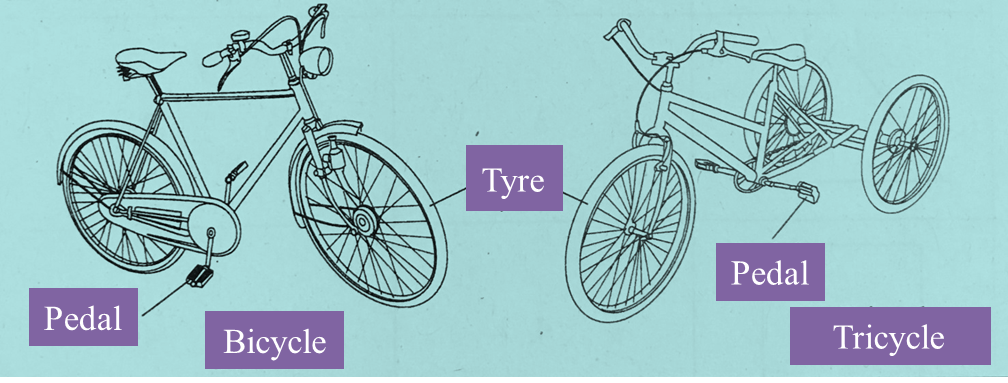# 6.4.6 Linear Equations, PT3 Focus Practice

Question 17:
Solving using matrix method is not allowed in this question.
Diagram shows a rectangular fish pond with the perimeter of 62 m.It is given that the length of the fish pond is 3 times its width.
Calculate the length, in m, of the fish pond.

Solution:
Given y + 4 = 3x
y = 3x – 4 …………. (1)

Perimeter = 62
2x + 2(y + 4) = 62
2x + 2y + 8 = 62
2x + 2y = 54
(÷2) x + y = 27 …………. (2)

Substitute (1) into (2):
x + 3x – 4 = 27
4x – 4 = 27
4x = 27 + 4
4x = 31
x = 7.75

From (1): y = 3x – 4
When x = 7.75
y = 3(7.75)4
y = 19.25

Length of the fish pond
= y + 4
= 19.25 + 4
= 23.25 m

Question 18:
Solution by matrix method is not allowed to answer this question.
Diagram shows a bicycle and a tricycle.Calculate the number of bicycles and the number of tricycles if there are 64 pedals and 74 tyres.

Solution:
Let bicycle = x and tricycle = y
So,
2x + 2y = 64 …………. (1)
2x + 3y = 74 …………. (2)
(2) – (1)
3y – 2y = 74 – 64
y = 10

When y = 10
From (2)
2x + 3(10) = 74
2x = 7430
2x = 44
x = 22

Thus, the number of bicycles are 22 and the number of tricycles are 10.